Courses

# ICAI Notes 3.2 - Theory of Cost CA Foundation Notes | EduRev

## CA Foundation : ICAI Notes 3.2 - Theory of Cost CA Foundation Notes | EduRev

The document ICAI Notes 3.2 - Theory of Cost CA Foundation Notes | EduRev is a part of the CA Foundation Course Economics for CA CPT.
All you need of CA Foundation at this link: CA Foundation

Learning Objectives

 understand the meaning of cost and various concepts of cost.
 understand cost function in the short run and in the long run.

2.0 COST ANALYSIS

Cost analysis refers to the study of behaviour of cost in relation to one or more production criteria, namely, size of output, scale of operations, prices of factors of production and other relevant economic variables. In other words, cost analysis is concerned with financial aspects of production relations as against physical aspects which were considered in production analysis. In order to have a clear understanding of the cost function it is important to understand various concepts of costs.

2.1 COST CONCEPTS

Accounting costs and economic costs : When an entrepreneur undertakes an act of production he has to pay prices for the factors which he employs for production. He thus pays, wages to workers employed, prices for the raw materials, fuel and power used, rent for the building he hires, and interest on the money borrowed for doing business. All these are included in his cost of production and are termed as accounting costs. Thus accounting costs take care of all the payments and charges made by the entrepreneur to the suppliers of various productive factors.

But it generally happens that an entrepreneur invests a certain amount of capital in his business. If the capital invested by the entrepreneur in his business had been invested elsewhere it would have earned certain amount of interest or dividend. Moreover, an entrepreneur devotes time to his own work of production and contributes his entrepreneurial and managerial ability to do business. Had he not set up his own business he would have sold his services to others for some positive amount of money. Accounting costs do not include these costs. These costs form a part of the economic cost.

Thus economic costs include :
(1) the normal return on money capital invested by the entrepreneur himself in his own business;
(2) the wages or salary not paid to the entrepreneur but could have been earned if the services had been sold somewhere else.

Likewise the monetary reward for all factors owned by the entrepreneur himself and employed by him in his own business are also considered a part of economic costs. Thus, accounting costs relate to those costs only which involve cash payments by the entrepreneur of the firm. Economic costs take into account these accounting costs but in addition, they also takes into account the amount of money the entrepreneur could have earned if he had invested his money and sold his own services and other factors in the next best alternative uses. Accounting costs are also called explicit costs whereas the cost of factors owned by the entrepreneur himself and employed in his own business are called implicit costs. Thus economic costs include both accounting costs and implicit costs. The concept of economic cost is important because an entrepreneur must cover his economic cost if he wants to earn normal profits and abnormal profits are over and above these normal profits. In other words, an entrepreneur is said to be earning profits (abnormal) only when his revenues are able to cover not only his explicit costs but also implicit costs.

Outlay costs and opportunity costs : Outlay costs involve actual expenditure of funds on, say, wages, material, rent, interest, etc. Opportunity cost, on the other hand, is concerned with the cost of foregone opportunity; it involves a comparison between the policy that was chosen and the policy that was rejected. For example, opportunity cost of using capital is the interest that it can earn in the next best use of equal risk.

A distinction between outlay costs and opportunity costs can be drawn on the basis of the nature of the sacrifice. Outlay costs involve financial expenditure at some time and hence are recorded in the books of account. Opportunity costs relate to sacrificed alternatives; they are not recorded in the books of account in general.

The opportunity cost concept is generally very useful, e.g., in a cloth mill which spins its own yarn, the opportunity cost of yarn to the weaving department is the price at which the yarn could be sold, for measuring profitability of the weaving operations. In long-term cost calculation also it is useful e.g., in calculating the cost of higher education, it is not the tuition fee and books but the earning foregone that should be taken into account.

Direct or traceable costs and indirect or non-traceable costs : Direct costs are costs that are readily identified and are traceable to a particular product, operation or plant. Even overhead can be direct as to a department; manufacturing costs can be direct to a product line, sales territory, customer class etc. We must know the purpose of cost calculation before considering whether a cost is direct or indirect.

Indirect costs are not readily identified nor visibly traceable to specific goods, services, operations, etc. but are nevertheless charged to the jobs or products in standard accounting practice. The economic importance of these costs is that these, even though not directly traceable to the product, may bear some functional relationship to production and may vary with output in some definite way.

Examples of such costs are electric power, the common costs incurred for general operation of business benefiting all products jointly.

Fixed and variable costs : Fixed or constant costs are not a function of output; they do not vary with output upto a certain level of activity. These costs require a fixed expenditure of funds irrespective of the level of output, e.g., rent, property taxes, interest on loans, depreciation when taken as a function of time and not of output. However, these costs also vary with the size of the plant and are a function of capacity. Therefore, fixed costs do not vary with the volume of output within a capacity level.

Fixed costs cannot be avoided. These costs are fixed so long as operations are going on. They can be avoided only when operations are completely closed down. We can call them as inescapable or uncontrollable costs. But there are some costs which will continue even after operations are suspended, as for example, the storing of old machines which cannot be sold in the market. Some of the fixed costs such as advertising, etc. are programmed fixed costs or discretionary expenses, because they depend upon the discretion of management whether to spend on these services or not.

Variable costs are costs that are a function of output in the production period. For example, wages and cost of raw materials are variable costs. Variable costs vary directly and sometimes proportionately with output. Over certain ranges of production they may vary less or more than proportionately depending on the utilization of fixed facilities and resources during production process.

2.2 COST FUNCTION

The cost function refers to the mathematical relation between cost of a product and the various determinants of costs. In cost function, the dependent variable is unit cost or total cost and the independent variables are the price of a factor, the size of the output or any other relevant phenomenon which has a bearing on cost such as technology, level of capacity utilization, efficiency and time period under consideration.

2.3 SHORT RUN TOTAL COSTS

Total, fixed and variable costs : There are some factors which can be easily adjusted with changes in the level of output. Thus a firm can readily employ more workers if it has to increase output. Similarly, it can purchase more raw material if it has to expand production. Such factors which can be easily varied with a change in the level of output are called variable factors. On the other hand, there are factors such as building, capital equipment, or top management team which cannot be so easily varied. It requires comparatively longer time to make changes in them. It takes time to install a new machinery. Similarly, it takes time to build a new factory. Such factors which cannot be readily varied and require a longer period to adjust are called fixed factors. Corresponding to the distinction between variable and fixed factors we distinguish between short run and long run periods of time. Short run is a period of time in which output can be increased or decreased by changing only the amount of variable factors, such as labour, raw material, etc. In the short run, quantities of fixed factors cannot be varied in accordance with changes in output. If the firm wants to increase output in the short run, it can do so only with the help of variable factors, i.e., by using more labour and/or by buying more raw material. Thus, short run is a period of time in which only variable factors can be varied, while the quantities of fixed factors remain unaltered. On the other hand, long run is a period of time in which the quantities of all factors may be varied. Thus all factors become variable in the long run.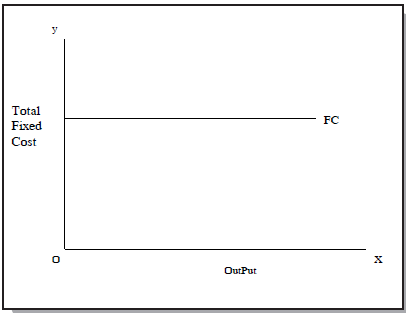Fig. 5 : Completely Fixed Cost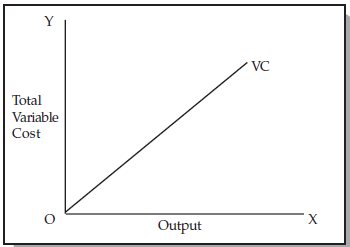Fig. 6 : Completely Variable Cost

Thus we find that fixed costs are those costs which are independent of output, i.e., they do not change with changes in output. These costs are a “fixed amount” which are incurred by a firm in the short run, whether the output is small or large. Even if the firm closes down for some time in the short run but remains in business, these costs have to be borne by it. Fixed costs include such charges as contractual rent, insurance fee, maintenance cost, property taxes, interest on capital employed, manager’s salary, watchman’s wages etc. Variable costs on the other hand are those costs which change with changes in output. These costs include payments such as wages of labour employed, prices of raw material, fuel and power used, transportation cost etc. If a firm shuts down for a short period, then it may not use variable factors of production and will not therefore incur any variable cost.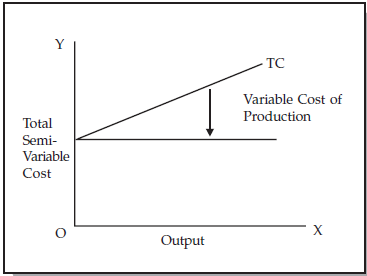Fig. 7 : Semi Variable Cost

There are some costs which are neither perfectly variable, nor absolutely fixed in relation to the changes in the size of output. They are known as semi-variable costs. Example: Electricity charges include both a fixed charge and a charge based on consumption.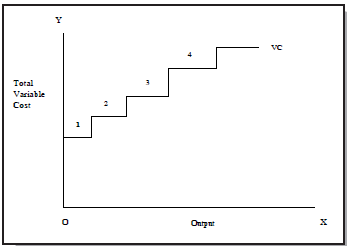Fig. 8 : A Stair-step Variable Cost

There are some costs which may increase in a stair-step fashion, i.e., they remain fixed over certain range of output; but suddenly jump to a new higher level when output goes beyond a given limit. Eg. Fixed salary of Foreman will have a sudden jump if another foreman is appointed when the output crosses a limit.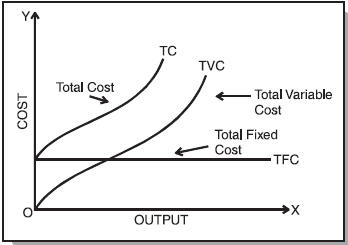Fig. 9 : Short run Total Cost Curves

Total cost of a business is thus the sum of total variable cost and total fixed cost or symbolically TC = TFC + TVC. We may also represent total cost, total variable cost and fixed cost diagramatically.

In the diagram, total fixed cost curve (TFC) is parallel to X-axis. This curve starts from the point on the Y-axis meaning thereby that fixed cost will be incurred even if the output is zero. On the other hand total variable cost curve rises upward showing thereby that as output increases, total variable cost also increases. This curve starts from the origin which shows that when the output is zero, variable costs are also nil. The total cost curve has been obtained by adding vertically total fixed cost curve and total variable cost curve.

Short run average cost

Average fixed cost (AFC) : AFC is the total fixed cost divided by the number of units of output produced. i.e. AFC =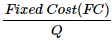where Q is the number of units produced. Thus average fixed cost is the fixed cost per unit of output. For example, a firm is producing with total fixed cost at Rs. 2,000/-. When output is 100 units, average fixed cost will be Rs. 20. And now if the output increases to 200 units, average fixed cost will be Rs. 10. Since total fixed cost is a constant
amount, average fixed cost will steadily fall as output increases. Therefore, if we draw average fixed cost curve, it will slope downwards throughout its length but not touch the X-axis as AFC can not be zero. (Fig. 10)

Average variable cost (AVC) : Average variable cost is the total variable cost divided by the number of units of output produced, i.e. AVC = TVC/Q where Q is the number of units produced. Thus average variable cost is variable cost per unit of output. Average variable cost normally falls as output increases from zero to normal capacity output due to occurrence of increasing returns. But beyond the normal capacity output, average variable cost will rise steeply because of the operation of diminishing returns (the concepts of increasing returns and diminishing returns have already been discussed earlier). If we draw average variable cost curve it will first fall, then reach a minimum and then rise again. (Fig. 10)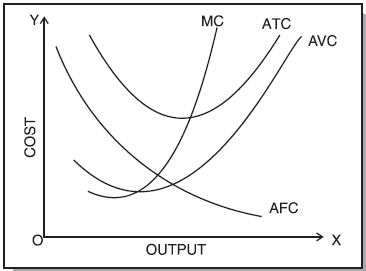Fig. 10 : Short run Average and Marginal Cost Curves

Average total cost (ATC) : Average total cost is a sum of average variable cost and average fixed cost. i.e., ATC = AFC + AVC. It is the total cost divided by the number of units produced. The behaviour of average total cost curve depends upon the behaviour of average variable cost curve and average fixed cost curve. In the beginning both AVC and AFC curves fall, therefore, the ATC curve will also fall sharply in the beginning. When AVC curve begins to rise, but AFC curve still falls steeply, ATC curve continues to fall. This is because during this stage the fall in AFC curve is greater than the rise in the AVC curve but as output increases further, there is a sharp rise in AVC which more than offsets the fall in AFC. Therefore, ATC curve first falls, reaches its minimum and then rises. Thus, the average total cost curve is “U” shape curve. (Fig. 10)

Marginal Cost : Marginal cost is the addition made to the total cost by production of an additional unit of output. In other words, it is the total cost of producing t units instead of t-1 units, where t is any given number. For example, if we are producing 5 units at a cost of Rs. 200 and now suppose 6th unit is produced and the total cost is Rs. 250, marginal cost is Rs. 250 - 200 i.e., Rs. 50. It is to be noted that marginal cost is independent of fixed cost. This is because fixed costs do not change with output. It is only the variable costs which change with a change in the level of output in the short run. Therefore, marginal cost is in fact due to the changes in variable costs. Symbolically margical cost can be written as :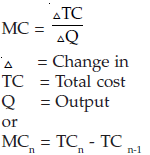Marginal cost curve falls as output increases in the beginning. It starts rising after a certain level of output. This happens because of the influence of the law of variable proportions. The fact that marginal product rises first, reaches a maximum and then declines ensures that the marginal cost curve of a firm declines first, reaches its minimum and then rises. In other words marginal cost curve of a firm is “U” shaped (see Figure 10). The behaviour of these costs has also been shown in Table 2.

Table 2 : Various Costs

 Units of  output Total Fixed Cost Total Variable Cost Total Cost Average Fixed Cost Average Variable Cost Average Total Cost Marginal Cost Per Unit 0 150 0 150 - - - - 6 150 50 200 25.0 8.33 33.33 50/6 = 8.33 16 150 100 250 9.83 6.25 15.63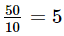29 150 150 300 5.17 5.17 10.34 50/13=3.85 44 150 200 350 3.41 4.55 7.95 50/15 = 3.33 55 150 250 400 2.73 4.55 7.27 50/11= 4.55 60 150 300 450 2.50 5.00 7.50 50/5 = 10

The above table shows that :
(i) Fixed cost does not change with increase in output upto a given range. Average fixed cost, therefore, comes down with every increase in output.
(ii) Variable cost increases but not necessarily in the same proportion as the increase in output. In the above case, average variable cost comes down gradually till 55 units are produced.
(iii) Marginal cost is the additional cost divided by addition units produced. This also comes gradually till 44 units are produced.

Relationship between Average Cost and Marginal Cost : The relationship between marginal cost and average cost is the same as that between any other marginal average quantities. The following are the points of relationship between the two phenomena.

(1) When average cost falls as a result of an increase in output, marginal cost is less than average cost.

(2) When average cost rises as a result of an increase in output, marginal cost is more than average cost.

(3) When average cost is minimum, marginal cost is equal to the average cost. In other words, marginal cost curve cuts average cost curve at its minimum point (i.e. optimum point).

Figure 10 and Table 2 confirm the above points of relationship.

2.4 LONG RUN AVERAGE COST CURVE

As stated above long run is a period of time during which the firm can vary all of its inputs - unlike short run in which some inputs are fixed and others are variable. In other words, whereas in the short run the firm is tied with a given plant, in the long run the firm moves from one plant to another; it can acquire a big plant if it wants to increase its output and a small plant if it wants to reduce its output. Long run cost of production is the least possible cost of producing any given level of output when all individual factors are variable. A long run cost curve depicts the functional relationship between output and the long run cost of production.

In order to understand how long run average cost curve is derived we consider three short run average cost curves as shown in Figure 11. These short run cost curves (SACs) are also called plant curves. In the short run the firm can be operating on any short run average cost curve given the size of the plant. Suppose that these are the only three plants which are technically possible. Given the size of the plant, the firm will be increasing or decreasing its output by changing the amount of the variable inputs. But in the long run, the firm chooses among the three possible sizes of plants as depicted by short run average curve (SAC1, SAC2, SAC3). In the long run, the firm will examine with which size of plants or on which short average cost curve it should operate to produce a given level of output so that total cost is minimum. It will be seen from the diagram that upto OB amount of output the firm will operate on the SAC1, though it could also produce with SAC2, because upto OB amount of output, the production on SAC1 results in lower cost than on SAC2. For example, if the level of output OA is produced with SAC1, it will cost AL per unit and if it is produced with SAC2 it will cost AH and we can see that AH is more than AL. Similarly, if the firm plans to produce an output which is larger than OB but less than OD then it will not be economical to produce on SAC1. For this, the firm will have to use SAC2. Similarly, the firm will use SAC3 for output larger than OD. It is thus clear that in the long run the firm has a choice in the employment of plant and it will employ that plant which yields minimum possible unit cost for producing a given output.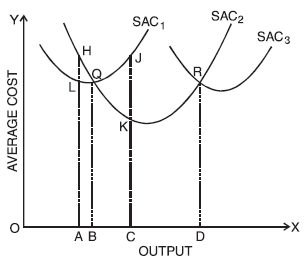Fig. 11 : Short run Average Cost Curves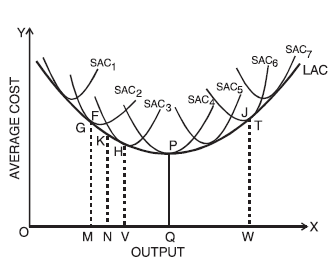Fig. 12 : Long run Average Cost Curves

Suppose now, the firm has a choice so that a plant can be varied by infinitely small gradations so that there are infinite number of plants corresponding to which there numerous average cost curves. In such a case the long run average cost curve will be a smooth curve enveloping all these short run average cost curves.

As shown in Figure 12 the long run average cost curve is so drawn as to be tangent to each of the short run average cost curves. Every point on the long run average cost curve will be a tangency point with some short run AC curve. If a firm desires to produce any particular output it then builds a corresponding plant and operate on the corresponding short run average cost curve. As shown in the figure, for producing OM the corresponding point on the LAC curve is G and the short run average cost curve SAC2 is tangent to the long run AC at this point. Thus if a firm desires to produce output OM, the firm will construct a plant corresponding to SAC2 and will operate on this curve at point G. Similarly, the firm will produce other levels of output choosing the plant which suits its requirements of lowest possible cost of production. It is clear from the figure that the large output can be produced at the lowest cost with the larger plant whereas smaller output can be produced at the lowest cost with smaller plants.

For example, to produce OM, the firm will be using SAC2 only; if it uses SACfor this, it will result in higher unit cost than SAC2. But larger output OV can be produced most economically with a larger plant represented by the SAC3. If we produce OV with the smaller plant it will result in higher unit similarly if we produce larger output with a smaller plant it will involve higher cost because of its limited capacity.

It is to be noted that LAC curve is not a tangent to the minimum points of the SAC curves. When the LAC curve is declining it is tangent to the falling portions of the short run cost curves and when the LAC curve is rising it is tangent to the rising portions of the short run cost curves. Thus for producing output less than “OQ” at the lowest possible unit cost the firm will construct the relevant plant and operate it at less than its full capacity, i.e., at less than its minimum average cost of production. On the other hand for output larger than OQ the firm will construct a plant and operate it beyond its optimum capacity. “OQ” is the optimum output. This is because “OQ” is being produced at the minimum point of LAC and corresponding SAC i.e., SAC4. Other plants are either used at less than their full capacity or more than their full capacity. Only SAC4 is being operated at the minimum point.

Long run average cost curve is often called a planning curve because a firm plans to produce any output in the long run by choosing a plant on the long run average cost curve corresponding to the given output. The long run average cost curve helps the firm in the choice of the size of the plant for producing a specific output at the least possible cost.

Explanation of the “U” shape of the long run average cost curve : As has been seen in the diagram LAC curve is a “U” shape curve. This shape of LAC curve depends upon the returns to scale. As discussed earlier, as the firm expands, returns to scale increase. After a range of constant returns to scale, the returns to scale finally decrease. On the same line, the LAC curve first declines and then finally rises. Increasing returns to scale cause fall in the long run average cost and decreasing returns to scale result in increase in long run average cost. Falling long run average cost and increasing economies to scale result from internal and external economies of scale and rising long run average cost and diminishing returns to scale from internal and external diseconomies of scale (economies of scale have been discussed earlier at the relevant place).

The long run average cost curve initially falls with increase in output and after a certain point it rises making a boat shape. Long-run Average cost (LAC) curve is also called the planning curve of the firm as it helps in choosing a plant on the decided level of output. The long-run avearge cost curve is called Envelope curve, because it envelopes or supports a family of short run average cost curves from below.

The above figure depicting long-run average cost curve is arrived at on the basis of traditional economic analysis. It is flattened ‘U’ shaped. This type of curve could exist only when the state of technology remains constant. But the empirical evidence shows that the state of technology changes in the long-run. Therefore, modern firms face ‘L-shaped’ cost curve than ‘u-shaped’. The L shaped cost curve is given below. According to the diagram, over AB range, the curve is perfectly flat. Over this range all sizes of plant have the same minimum cost.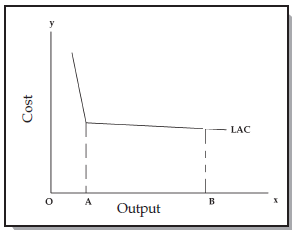Fig. 13 : Long-run Average Cost Curve

SUMMARY

The relation between cost and output is called “Cost Function”. Cost function of a firm depends upon its production function and the prices of factors of production.

In cost analysis a number of cost concepts are employed like accounting costs (costs which are accounted for), economic costs (costs which are accounted for plus costs which are not incurred but would have been incurred but for the employment of self services by the entrepreneurs), direct costs (costs which can be directly traced to a function or product), indirect costs (costs which cannot be related directly to product or function) and so on. Economists are generally interested in two types of cost functions, the short run cost function and the long run cost function and accordingly derive the short run and long run cost curves. Related to short run and long run periods we have fixed cost concept and variable cost concept.

Fixed cost are not a function of output and they are fixed in the short run. Variable costs, on the other hand, are variable with the level of output. In the long run all costs become variable. Cost of production is the most important force governing the supply of a product. It should be noted that it is assumed that for each level of output, the firm chooses the least cost combination of factors.

,

,

,

,

,

,

,

,

,

,

,

,

,

,

,

,

,

,

,

,

,

;